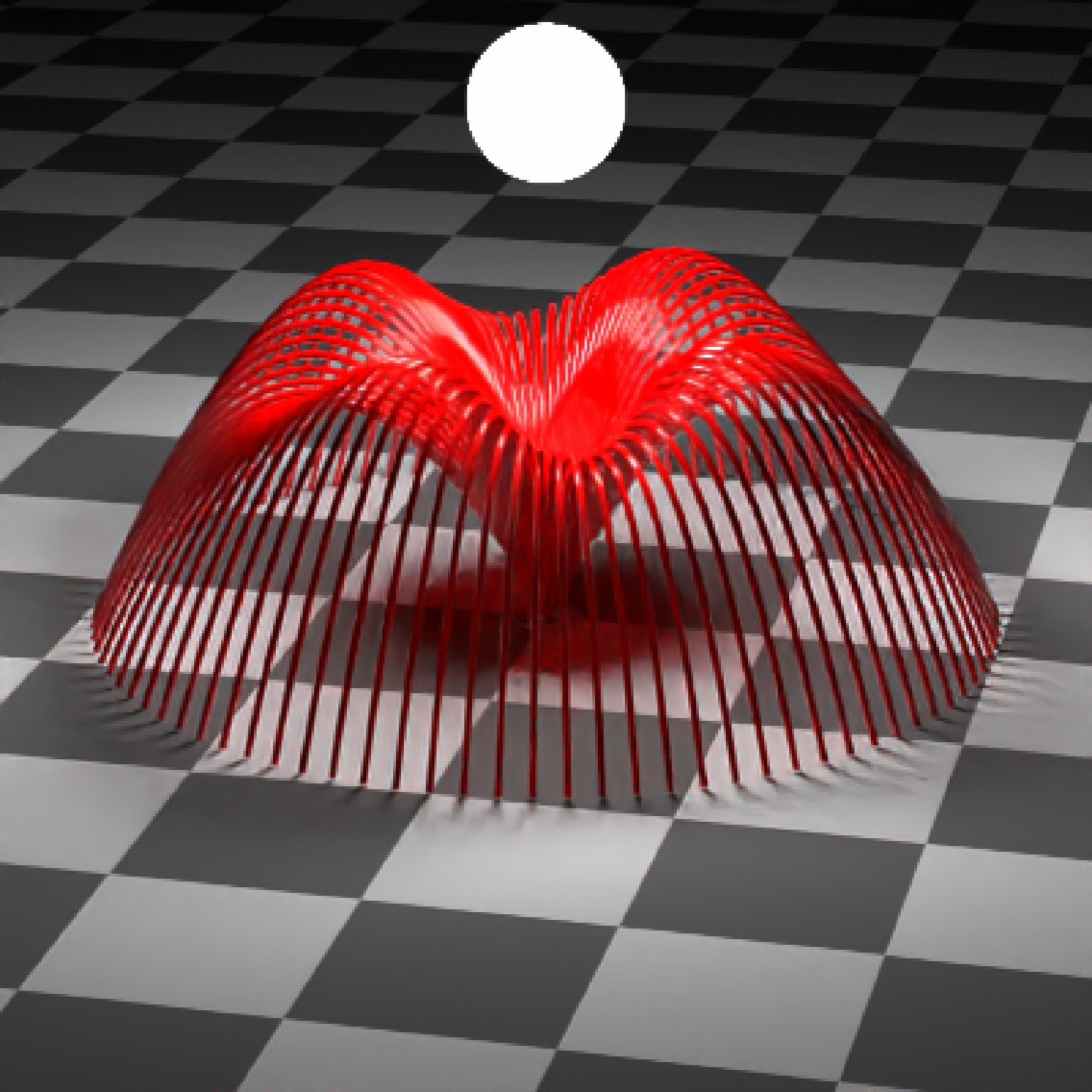Bezier curve, defined by 4 control points.

bezier_curve(
p1 = c(0, 0, 0),
p2 = c(-1, 0.33, 0),
p3 = c(1, 0.66, 0),
p4 = c(0, 1, 0),
x = 0,
y = 0,
z = 0,
width = 0.1,
width_end = NA,
u_min = 0,
u_max = 1,
type = "cylinder",
normal = c(0, 0, -1),
normal_end = NA,
material = diffuse(),
angle = c(0, 0, 0),
order_rotation = c(1, 2, 3),
velocity = c(0, 0, 0),
flipped = FALSE,
scale = c(1, 1, 1)
)

## Arguments

p1 Default c(0,0,0). First control point. Can also be a list of 4 length-3 numeric vectors or 4x3 matrix/data.frame specifying the x/y/z control points. Default c(-1,0.33,0). Second control point. Default c(1,0.66,0). Third control point. Default c(0,1,0). Fourth control point. Default 0. x-coordinate offset for the curve. Default 0. y-coordinate offset for the curve. Default 0. z-coordinate offset for the curve. Default 0.1. Curve width. Default NA. Width at end of path. Same as width, unless specified. Default 0. Minimum parametric coordinate for the curve. Default 1. Maximum parametric coordinate for the curve. Default cylinder. Other options are flat and ribbon. Default c(0,0,-1). Orientation surface normal for the start of ribbon curves. Default NA. Orientation surface normal for the start of ribbon curves. If not specified, same as normal. Default diffuse.The material, called from one of the material functions diffuse, metal, or dielectric. Default c(0, 0, 0). Angle of rotation around the x, y, and z axes, applied in the order specified in order_rotation. Default c(1, 2, 3). The order to apply the rotations, referring to "x", "y", and "z". Default c(0, 0, 0). Velocity of the cube. Default FALSE. Whether to flip the normals. Default c(1, 1, 1). Scale transformation in the x, y, and z directions. If this is a single value, number, the object will be scaled uniformly. Note: emissive objects may not currently function correctly when scaled.

## Value

Single row of a tibble describing the cube in the scene.

## Examples

#Generate the default curve:
# \donttest{
generate_studio(depth=-0.1) %>%
material=light(intensity=200, spotlight_focus = c(0,0.5,0)))) %>%
render_scene(clamp_value = 10, lookat = c(0,0.5,0), fov=13,
samples=500, sample_method = "stratified")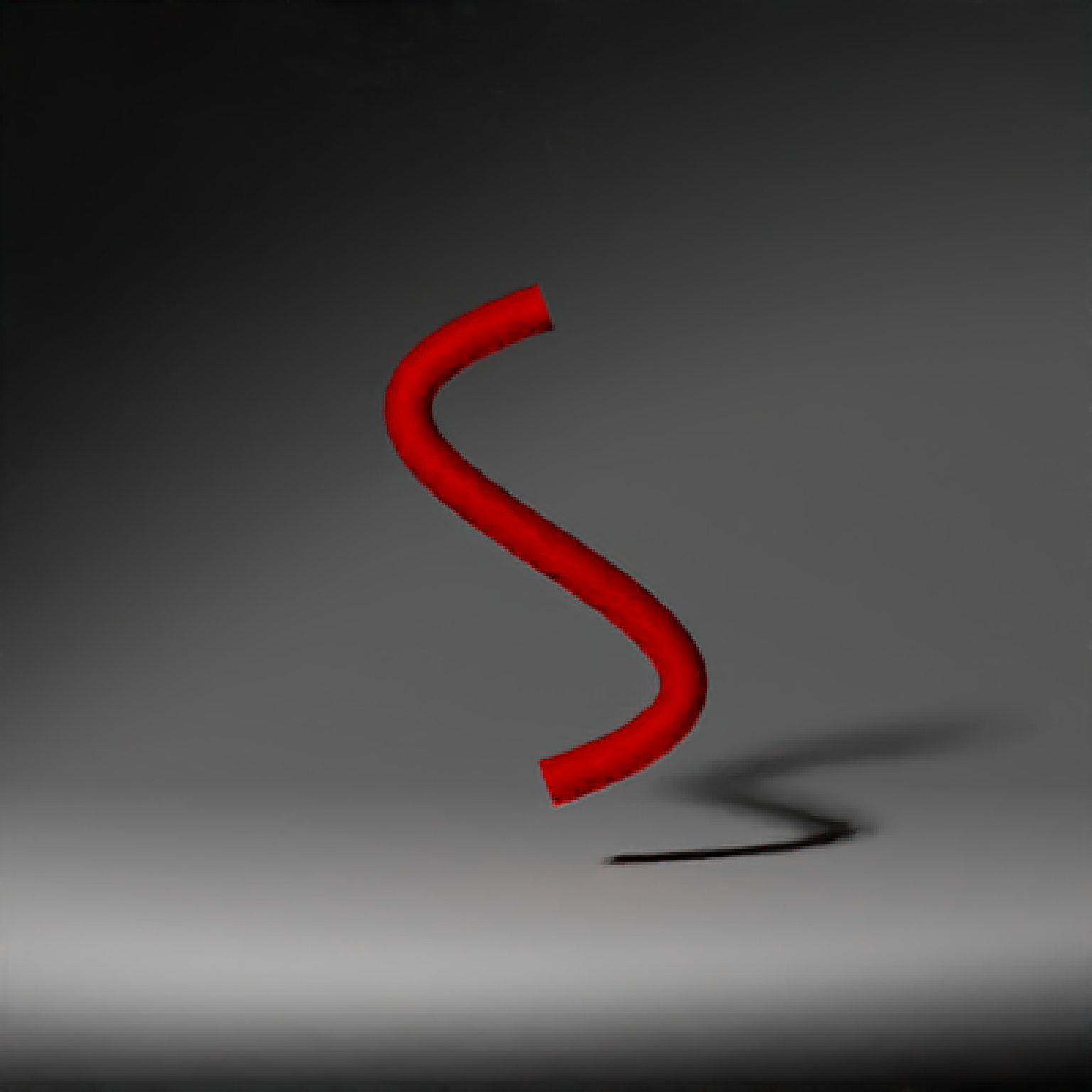#Change the control points to change the direction of the curve. Here, we place spheres
#at the control point locations.
generate_studio(depth=-0.1) %>%
material=light(intensity=200, spotlight_focus = c(0,0.5,0)))) %>%
render_scene(clamp_value = 10, lookat = c(0,0.5,0), fov=15,
samples=500, sample_method = "stratified")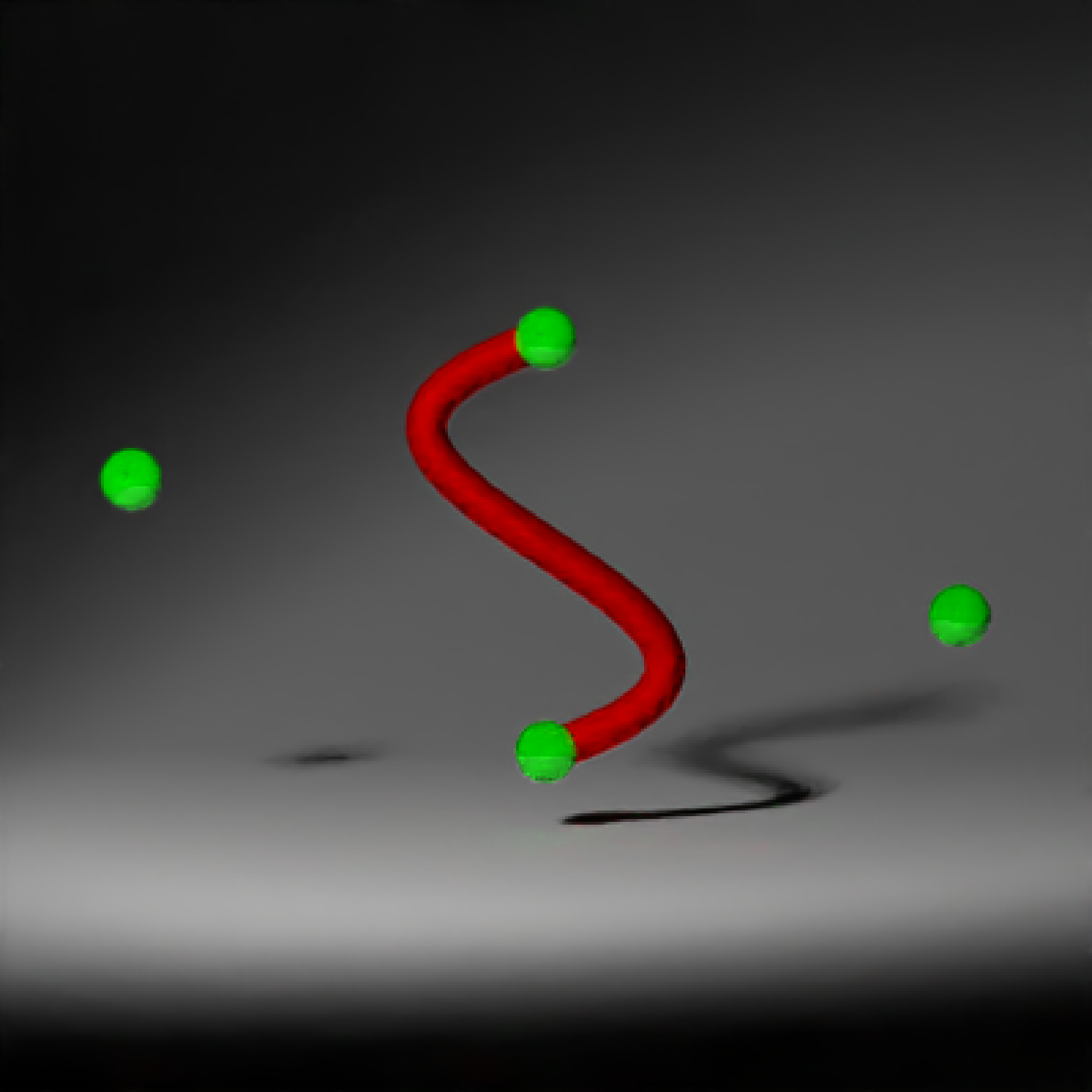#We can make the curve flat (always facing the camera) by setting the type to flat
generate_studio(depth=-0.1) %>%
material=light(intensity=200, spotlight_focus = c(0,0.5,0)))) %>%
render_scene(clamp_value = 10, lookat = c(0,0.5,0), fov=13,
samples=500, sample_method = "stratified")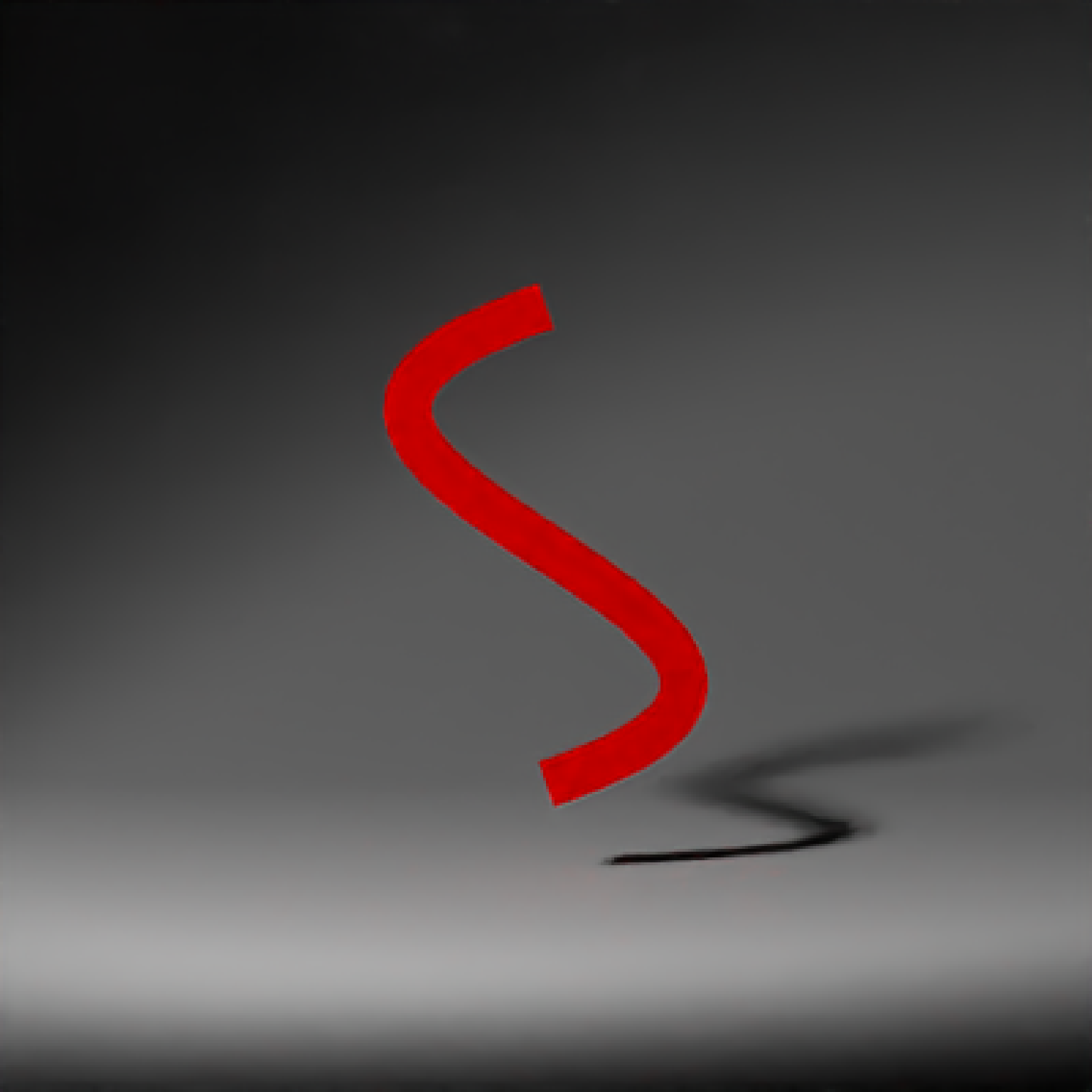#We can also plot a ribbon, which is further specified by a start and end orientation with
#two surface normals.
generate_studio(depth=-0.1) %>%
p1 = c(0,0,0), p2 = c(0,0.33,0), p3 = c(0,0.66,0), p4 = c(0.3,1,0),
normal_end = c(0,0,1),
material=glossy(color="red"))) %>%
material=light(intensity=200, spotlight_focus = c(0,0.5,0)))) %>%
render_scene(clamp_value = 10, lookat = c(0,0.5,0), fov=13,
samples=500, sample_method = "stratified")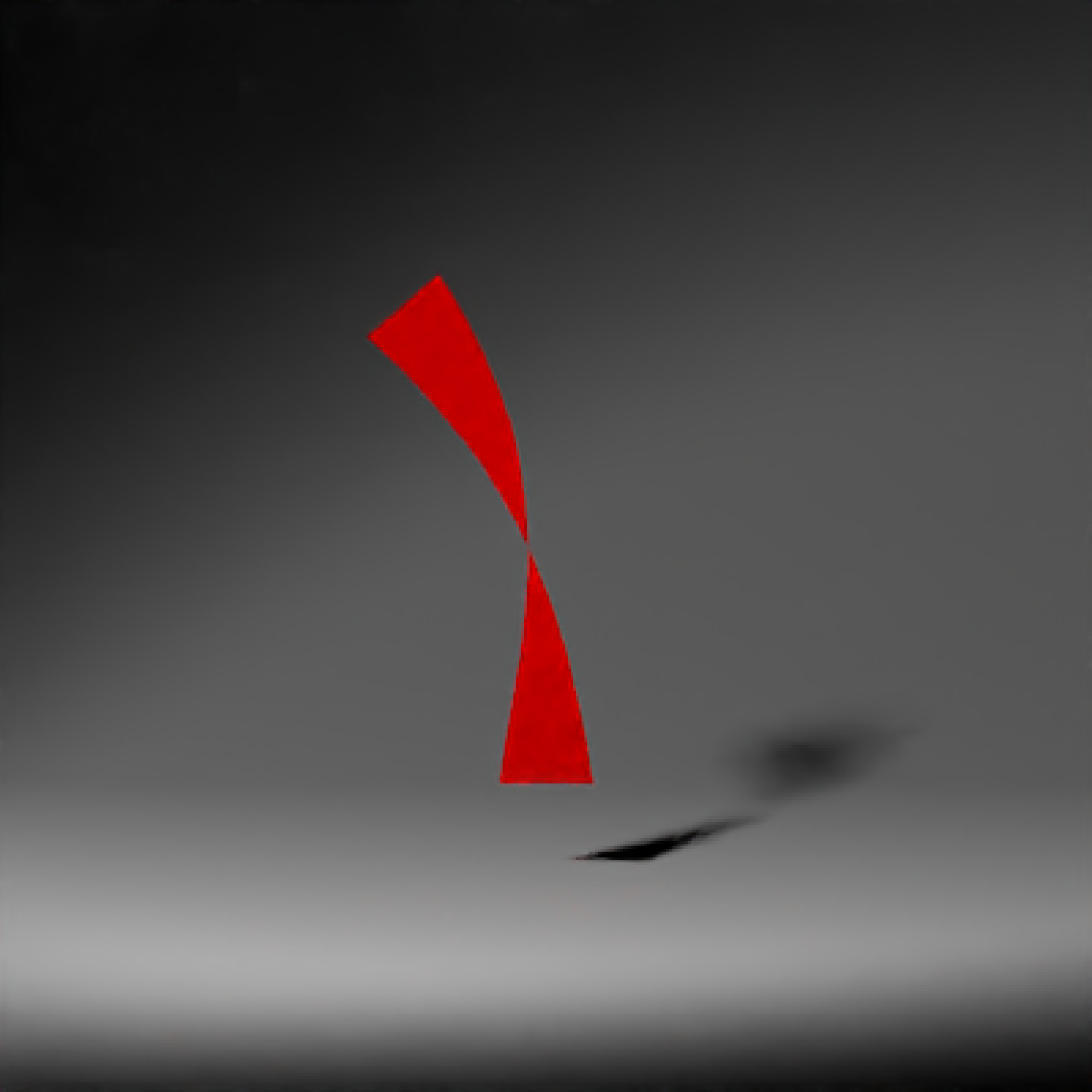#Create a single curve and copy and rotate it around the y-axis to create a wavy fountain effect:
scene_curves = list()
for(i in 1:90) {
scene_curves[[i]] = bezier_curve(p1 = c(0,0,0),p2 = c(0,5-sinpi(i*16/180),2),
p3 = c(0,5-0.5 * sinpi(i*16/180),4),p4 = c(0,0,6),
angle=c(0,i*4,0), type="cylinder",
width = 0.1, width_end =0.1,material=glossy(color="red"))
}
all_curves = do.call(rbind, scene_curves)
generate_ground(depth=0,material=diffuse(checkercolor="grey20")) %>%
lookat=c(0,1,0), fov=15, clamp_value = 10)